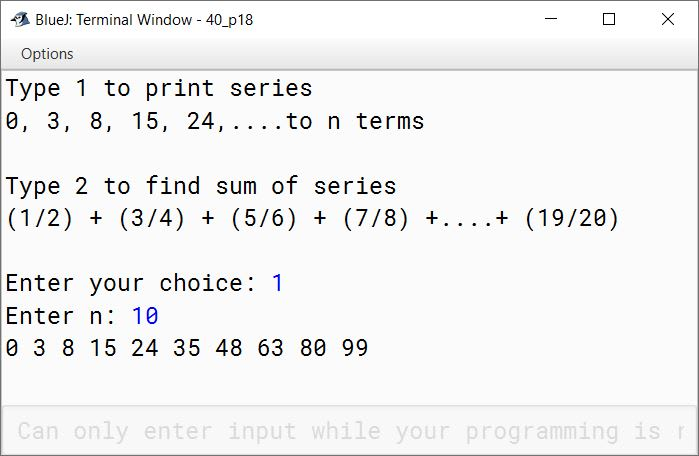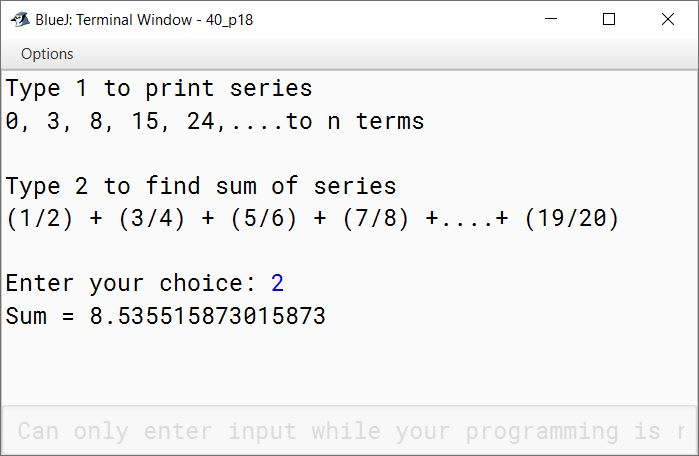# Solved 2011 Question Paper ICSE Class 10 Computer Applications

## Section A

#### Question 1

(a) What is the difference between an object and a class?

A class is a blue print that represents a set of objects that share common characteristics and behaviour whereas an object is a specific instance of a class having a specific identity, specific characteristics and specific behaviour.

(b) What does the token 'keyword' refer to in the context of Java? Give an example for keyword.

Keywords are reserved words that have a special meaning for the Java compiler. Java compiler reserves these words for its own use so Keywords cannot be used as identifiers. An example of keyword is class.

(c) State the difference between entry controlled loop and exit controlled loop.

Entry controlled loopExit controlled loop
It checks the condition at the time of entry. Only if the condition is true, the program control enters the body of the loop.It checks the condition after executing its body. If the condition is true, loop will perform the next iteration otherwise program control will move out of the loop.
Loop does not execute at all if the condition is false.The loop executes at least once even if the condition is false.
Example: for and while loopsExample: do-while loop

(d) What are the two ways of invoking functions?

Two ways of invoking functions are:

1. Pass by value.
2. Pass by reference.

(e) What is the difference between / and % operators?

/%
Division operatorModulus operator
Returns the quotient of division operationReturns the remainder of division operation
Example: int a = 5 / 2; Here a will get the value of 2 which is the quotient of this division operationExample: int b = 5 % 2; Here b will get the value of 1 which is the remainder of this division operation

#### Question 2

(a) State the total size in bytes, of the arrays a of char data type and p of float data type.

Size of char a is 4 × 2 = 8 Bytes.
Size of float p is 4 × 4 = 16 Bytes.

(b) (i) Name the package that contains Scanner class.
(ii) Which unit of the class gets called, when the object of the class is created?

(i) java.util
(ii) Constructor

(c) Give the output of the following:

String n = "Computer Knowledge";
String m = "Computer Applications";
System.out.println(n.substring(0, 8).concat(m.substring(9)));
System.out.println(n.endsWith("e"));

##### Output
ComputerApplications
true

##### Explanation

n.substring(0,8) returns the substring of n starting at index 0 till 7 (i.e. 8 - 1 = 7) which is "Computer". m.substring(9) returns the substring of m starting at index 9 till the end of the string which is "Applications". concat() method joins "Computer" and "Applications" together to give the output as ComputerApplications.

(d) Write the output of the following:

1. System.out.println(Character.isUpperCase('R'));
2. System.out.println(Character.toUpperCase('j'));

1. true
2. J

(e) What is the role of keyword void in declaring functions?

The keyword 'void' signifies that the function doesn't return a value to the calling function.

#### Question 3

(a) Analyze the following program segment and determine how many times the loop will be executed and what will be the output of the program segment?

int p = 200;
while(true){
if(p < 100)
break;
p = p - 20;
}
System.out.println(p);

##### Output
80


The loop executes 6 times

##### Explanation
pRemarks
200Initial Value
1801st Iteration
1602nd Iteration
1403rd Iteration
1204th Iteration
1005th Iteration
806th Iteration. Now p < 100 becomes true so break statement is executed terminating the loop.

(b) What will be the output of the following code?

(i)

int k = 5, j = 9;
k += k++ - ++j + k;
System.out.println("k = " + k);
System.out.println("j = " + j);

(ii)

double b = -15.6;
double a = Math.rint(Math.abs(b));
System.out.println("a = " + a);

(i)

##### Output
k = 6
j = 10

##### Explanation

k += k++ - ++j + k
⇒ k = 5 + (5 - 10 + 6)
⇒ k = 5 + 1
⇒ k = 6

++j increments j to 10

(ii)

##### Output
16.0

##### Explanation

Math.abs(-15.6) gives 15.6. Math.rint(15.6) gives 16.0.

Constructor overloading is a technique in Java through which a class can have more than one constructor with different parameter lists. The different constructors of the class are differentiated by the compiler using the number of parameters in the list and their types. For example, the Rectangle class below has two constructors:

class Rectangle {
int length;

//Constructor 1
public Rectangle() {
length = 0;
}

//Constructor 2
public Rectangle(int l, int b) {
length = l;
}

public static void main(String[] args) {
//Constructor 1 called
Rectangle obj1 = new Rectangle();

//Constructor 2 called
Rectangle obj2 = new Rectangle(10, 15);
}
}

(d) Give the prototype of a function search which receives a sentence 'sent' and word 'w' and returns 1 or 0.

int search(String sent, String w)

(e) Write an expression in Java for:

$z = \dfrac{5x^3 + 2y}{x + y}$

z = (5 * x * x * x + 2 * y) / (x + y)

(f) Write a statement each to perform the following tasks on a string:

1. Find and display the position of the last space in a string s.
2. Convert a number stored in a string variable x to double data type.

1. System.out.println(s.lastIndexOf(" "));
2. double num = Double.parseDouble(x);

(g) Name the keyword that:

1. informs that an error has occurred in an input/output operation.
2. distinguishes between instance variable and class variable.

1. throws IOException
2. static

(h) What are library classes? Give an example.

Java Library classes are a set of predefined classes in the form of packages that are provided to the programmer as part of Java installation. Library classes simplify the job of programmers by providing built-in methods for common and non-trivial tasks like taking input from user, displaying output to user, etc. For example, System class in java.lang package of Java Library classes provides the print() and println() methods for displaying output to user.

(i) Write one difference between Linear Search and Binary Search.

Linear search works on unsorted as well as sorted arrays whereas Binary search works on only sorted arrays.

## Section B

#### Question 4

Define a class called 'Mobike' with the following specifications:

Data MembersPurpose
int bnoTo store the bike number
int phnoTo store the phone number of the customer
String nameTo store the name of the customer
int daysTo store the number of days the bike is taken on rent
int chargeTo calculate and store the rental charge
Member MethodsPurpose
void input()To input and store the details of the customer
void compute()To compute the rental charge
void display()To display the details in the given format

The rent for a mobike is charged on the following basis:

DaysCharge
For first five days₹500 per day
For next five days₹400 per day
Rest of the days₹200 per day

Output:

Bike No.    Phone No.   Name    No. of days     Charge
xxxxxxx     xxxxxxxx    xxxx        xxx         xxxxxx       

import java.util.Scanner;

public class Mobike
{
private int bno;
private int phno;
private int days;
private int charge;
private String name;

public void input() {
Scanner in = new Scanner(System.in);
System.out.print("Enter Customer Name: ");
name = in.nextLine();
System.out.print("Enter Customer Phone Number: ");
phno = in.nextInt();
System.out.print("Enter Bike Number: ");
bno = in.nextInt();
System.out.print("Enter Number of Days: ");
days = in.nextInt();
}

public void compute() {
if (days <= 5)
charge = days * 500;
else if (days <= 10)
charge = (5 * 500) + ((days - 5) * 400);
else
charge = (5 * 500) + (5 * 400) + ((days - 10) * 200);
}

public void display() {
System.out.println("Bike No.\tPhone No.\tName\tNo. of days \tCharge");
System.out.println(bno + "\t" + phno + "\t" + name + "\t" + days
+ "\t" + charge);
}

public static void main(String args[]) {
Mobike obj = new Mobike();
obj.input();
obj.compute();
obj.display();
}
}
##### Output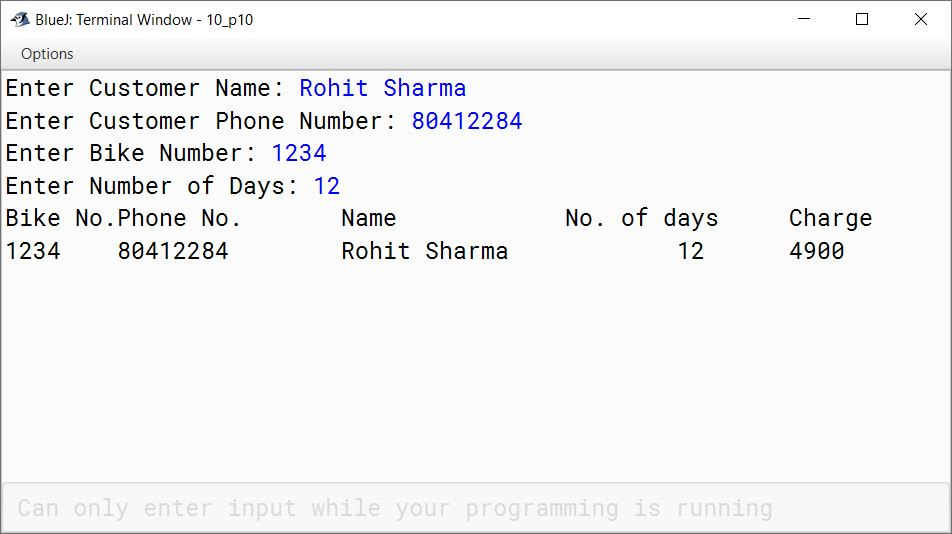#### Question 5

Write a program to input and sort the weight of ten people. Sort and display them in descending order using the selection sort technique.

import java.util.Scanner;

public class KboatSelectionSort
{
public static void main(String args[]) {
Scanner in = new Scanner(System.in);
double weightArr[] = new double;
System.out.println("Enter weights of 10 people: ");
for (int i = 0; i < 10; i++) {
weightArr[i] = in.nextDouble();
}

for (int i = 0; i < 9; i++) {
int idx = i;
for (int j = i + 1; j < 10; j++) {
if (weightArr[j] > weightArr[idx])
idx = j;
}

double t = weightArr[i];
weightArr[i] = weightArr[idx];
weightArr[idx] = t;
}

System.out.println("Sorted Weights Array:");
for (int i = 0; i < 10; i++) {
System.out.print(weightArr[i] + " ");
}
}
}
##### Output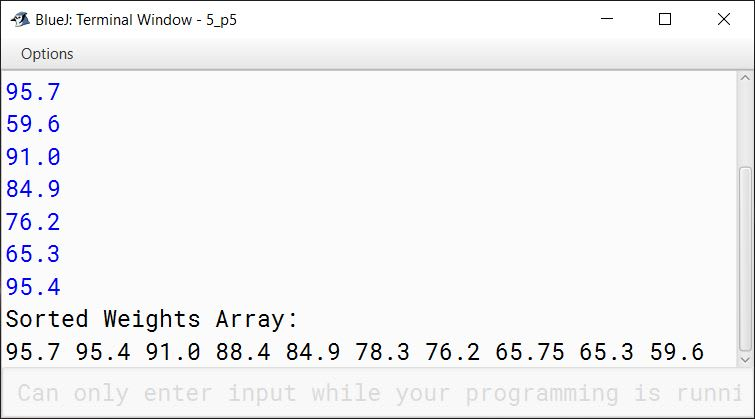#### Question 6

Write a program to input a number and print whether the number is a special number or not.

(A number is said to be a special number, if the sum of the factorial of the digits of the number is same as the original number).

Example:
145 is a special number, because 1! + 4! + 5! = 1 + 24 + 120 = 145.
(Where ! stands for factorial of the number and the factorial value of a number is the product of all integers from 1 to that number, example 5! = 1 * 2 * 3 * 4 * 5 = 120)

import java.util.Scanner;

public class KboatSpecialNum
{
public static int fact(int y) {
int f = 1;
for (int i = 1; i <= y; i++) {
f *= i;
}
return f;
}

public static void main(String args[]) {
Scanner in = new Scanner(System.in);
System.out.print("Enter number: ");
int num = in.nextInt();

int t = num;
int sum = 0;
while (t != 0) {
int d = t % 10;
sum += fact(d);
t /= 10;
}

if (sum == num)
System.out.println(num + " is a special number");
else
System.out.println(num + " is not a special number");

}
}
##### Output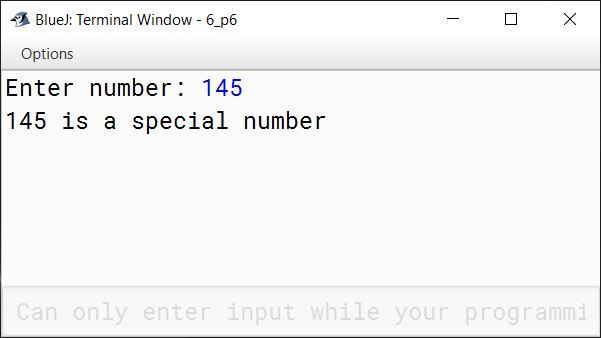#### Question 7

Write a program to accept a word and convert it into lower case, if it is in upper case. Display the new word by replacing only the vowels with the letter following it.
Sample Input: computer
Sample Output: cpmpvtfr

import java.util.Scanner;

public class KboatVowelReplace
{
public static void main(String args[]) {
Scanner in = new Scanner(System.in);
System.out.print("Enter a word: ");
String str = in.nextLine();
str = str.toLowerCase();
String newStr = "";
int len = str.length();

for (int i = 0; i < len; i++) {
char ch = str.charAt(i);

if (str.charAt(i) == 'a' ||
str.charAt(i) == 'e' ||
str.charAt(i) == 'i' ||
str.charAt(i) == 'o' ||
str.charAt(i) == 'u') {

char nextChar = (char)(ch + 1);
newStr = newStr +  nextChar;

}
else {
newStr = newStr + ch;
}
}

System.out.println(newStr);
}
}
##### Output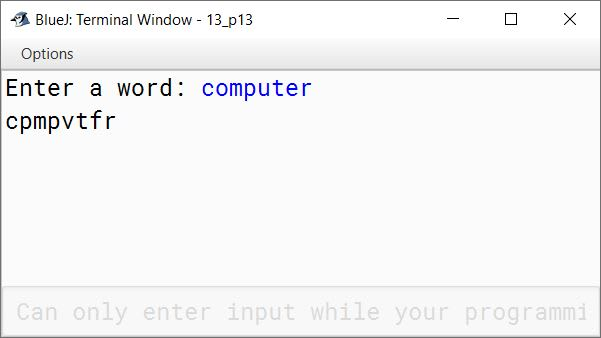#### Question 8

Design a class to overload a function compare( ) as follows:

1. void compare(int, int) — to compare two integers values and print the greater of the two integers.
2. void compare(char, char) — to compare the numeric value of two characters and print with the higher numeric value.
3. void compare(String, String) — to compare the length of the two strings and print the longer of the two.

import java.util.Scanner;

public class KboatCompare
{
public void compare(int a, int b) {

if (a > b) {
System.out.println(a);
}
else {
System.out.println(b);
}

}

public void compare(char a, char b) {
int x = (int)a;
int y = (int)b;

if (x > y) {
System.out.println(a);
}
else {
System.out.println(b);
}

}

public void compare(String a, String b) {

int l1 = a.length();
int l2 = b.length();

if (l1 > l2) {
System.out.println(a);
}
else {
System.out.println(b);
}

}

public static void main(String args[]) {
Scanner in = new Scanner(System.in);
KboatCompare obj = new KboatCompare();

System.out.print("Enter first integer: ");
int n1 = in.nextInt();
System.out.print("Enter second integer: ");
int n2 = in.nextInt();
obj.compare(n1, n2);

System.out.print("Enter first character: ");
char c1 = in.next().charAt(0);
System.out.print("Enter second character: ");
char c2 = in.next().charAt(0);
in.nextLine();
obj.compare(c1, c2);

System.out.print("Enter first string: ");
String s1 = in.nextLine();
System.out.print("Enter second string: ");
String s2 = in.nextLine();
obj.compare(s1, s2);
}
}
##### Output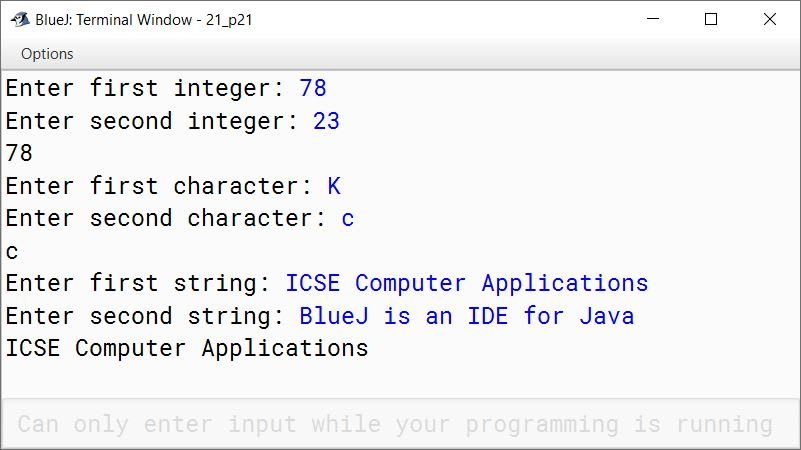#### Question 9

Write a menu driven program to perform the following tasks by using Switch case statement:

(a) To print the series:
0, 3, 8, 15, 24, ............ to n terms. (value of 'n' is to be an input by the user)

(b) To find the sum of the series:
S = (1/2) + (3/4) + (5/6) + (7/8) + ........... + (19/20)

import java.util.Scanner;

{
public static void main(String args[]) {
Scanner in = new Scanner(System.in);
System.out.println("Type 1 to print series");
System.out.println("0, 3, 8, 15, 24,....to n terms");
System.out.println();
System.out.println("Type 2 to find sum of series");
System.out.println("(1/2) + (3/4) + (5/6) + (7/8) +....+ (19/20)");
System.out.println();
int choice = in.nextInt();

switch (choice) {
case 1:
System.out.print("Enter n: ");
int n = in.nextInt();
for (int i = 1; i <= n; i++)
System.out.print(((i * i) - 1) + " ");
System.out.println();
break;

case 2:
double sum = 0;
for (int i = 1; i <= 19; i = i + 2)
sum += i / (double)(i + 1);
System.out.println("Sum = " + sum);
break;

default:
System.out.println("Incorrect Choice");
break;
}
}
}
##### Output